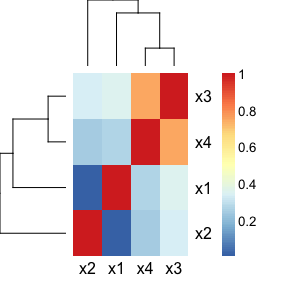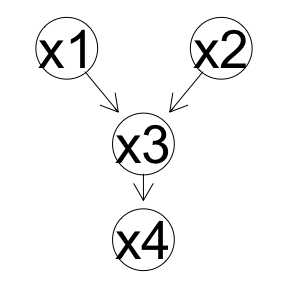A quick introduction to iTOP

2018-06-13

iTOP is the R package accompanying our publication on the inference of topologies of relationships between datasets, such as multi-omics and phenotypic data recorded on the same samples (Aben et al., 2018, doi.org/10.1101/293993). We based this methodology on the RV coefficient, a measure of matrix correlation, which we have extended for partial matrix correlations and binary data.

In this vignette, we will provide code examples for inferring a topology of continuous value datasets first. Subsequently, we will consider inferring a topology of a mix of continuous and binary value data types, using data type specific configuration matrices.

Inferring a topology using continuous value datasets

Artificial data

Let us first create some simple artificial data. Note that each dataset needs to describe the same set of samples, but not necessarily the same set of features. However, for simplicity, we also use the same number of features for all datasets in this example.

Matrix correlations

We will compare the datasets $$x_1$$, $$x_2$$, $$x_3$$ and $$x_4$$ with each other using matrix correlations. To this end, we will:

• make sure all datasets have the same set of samples in the same order, so that they can be compared (we have now created the data such that this is already the case, but it is good practice to check anyway);
• convert the datasets to configuration matrices (i.e. similarity matrices); and finally
• compute the matrix correlation between each pair of datasets, which we will save in the correlation matrix $$cors$$.

The correlation matrix $$cors$$ gives us an initial idea of how the datasets are related. For example, the matrix correlation $$RV(x_1,x_2)$$ is nearly zero, suggesting that they are not directly related.Statistical inference for matrix correlations

Such statements can be tested statistically. Here, we used a permutation test to obtain p-values and a bootstrapping procedure to obtain confidence intervals. Indeed, we see that there is no significant relation between $$x_1$$ and $$x_2$$.

##  0.01009952
##        2.5%       97.5%
## -0.03642779  0.05854942
##  0.521

Statistical inference for partial matrix correlations

We can easily extend such questions to partial matrix correlations. For example, we find that $$RV(x_1, x_4 | x_3)$$ is not significantly different from zero, implying that all information that is shared between $$x_1$$ and $$x_4$$ is contained in $$x_3$$.

##  0.009119728
##  0.506
##        2.5%       97.5%
## -0.03654138  0.05806329

On the other hand, we find that $$RV(x_3, x_4 | x_1, x_2)$$ is significantly different from zero, implying that $$x_3$$ and $$x_4$$ share information that is not present in $$x1$$ and $$x_2$$.

##  0.6984827
##  0
##      2.5%     97.5%
## 0.6731566 0.7213591

Inferring a topology of interactions between datasets

Of course, the number of possible partial matrix correlations to consider is very large. To summarize all of these, we can use the PC algorithm to construct a topology of interactions between datasets. In this example, the algorithm was even able to infer the causality between the datasets!

NB: if you have trouble installing the pcalg package, you can use the following code to do so (as sometimes installing dependencies from Bioconductor seems to fail for this package). On some Linux systems, we also needed to install some additional libraries:

• sudo apt-get install libv8-3.14-dev
• sudo apt-get install libgmp3-devInferring a topology using data type specific configuration matrices

Artificial data

Consider again the same artificial data as above, but now with a binary version of $$x1$$ and $$x4$$.

Computing data type specific configuration matrices

In the regular RV coefficient, the configuration matrices are determined using inner product as a similarity measure (this is also the default setting in this package). Here, we will set this similarity measure to Jaccard similarity for $$x_1$$ and $$x_4$$.

Of course, it is possible to use other similarity measures: simply set similarity_fun[[i]] to the function of your choice. This function should take two vectors (each representing a sample) as an input and should return a single number (representing the similarity between those two samples) as an output. We recommend using a similarity measure that results in symmetric and positive semi-definite configuration matrices. When using new similarity functions, it is also be worthwhile to experiment with the center parameter in compute.config.matrices(). For inner product similarity and Jaccard similarity, we recommend using centering (of note, in order to center binary data, we center the configuration matrices using kernel centering, for details see our manuscript: Aben et al., 2018, doi.org/10.1101/293993). However, for some other similarity measures, centering may not be beneficial (for example, because the measure itself is already centered, such as in the case of Pearson correlation).

Alternatively, if it is hard to represent the configuration matrix as a function of its samples, it is also possible to set the entire configuration matrix at once. Once again, we recommend the use of symmetric and positive semi-definite configuration matrices, and we suggest careful assessment of whether centering is required.

Using the data type specific configuration matrices

Once the configuration matrices have been computed, all other tools from the package can be used as before.